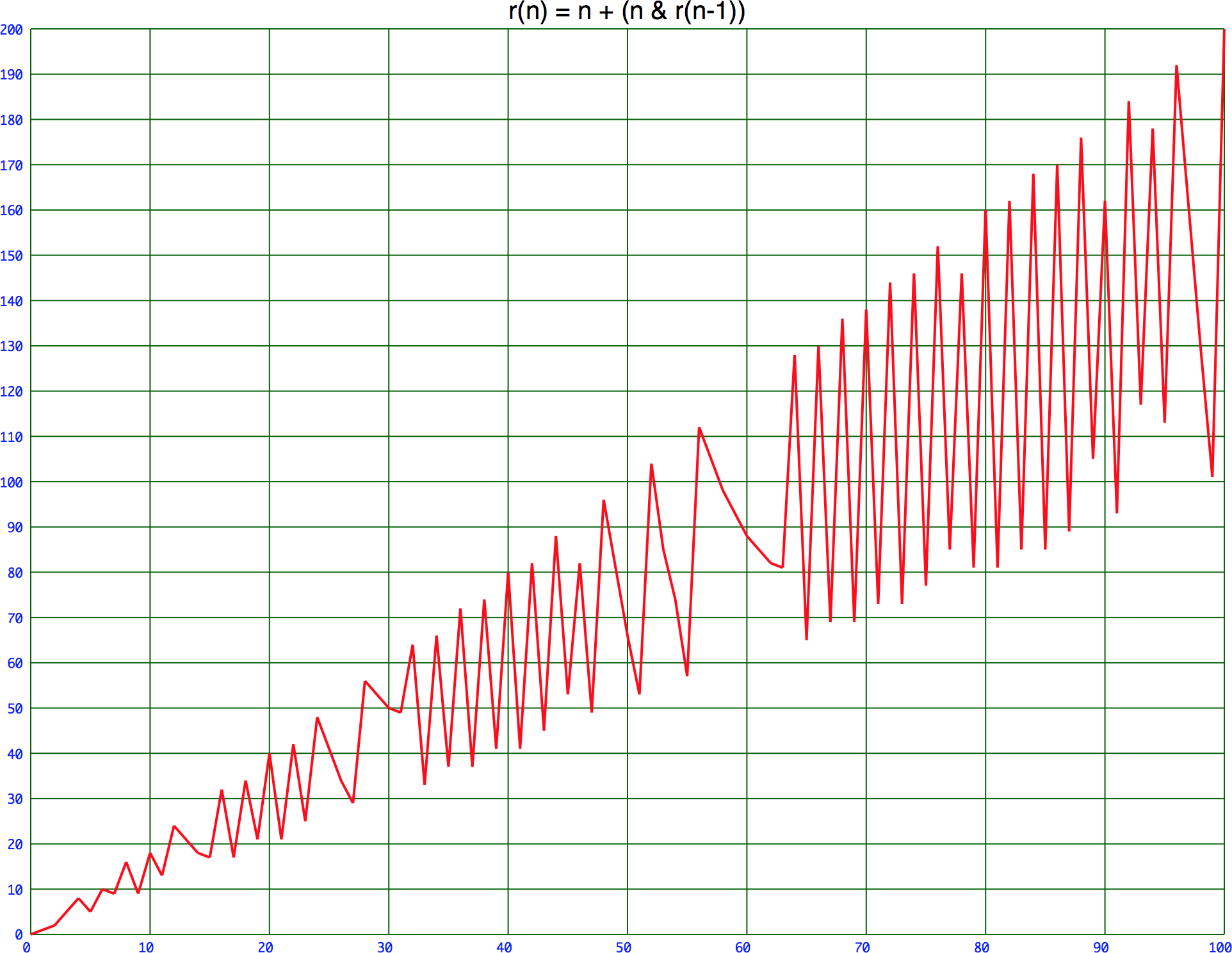## Recursive functions without a base case

19th December 2019

In an earlier post Recursion without a base case I wrote about the fact that it's possible to define a recursive function without an explicit base case. I've recently been exploring some other recursive functions of this sort.

### What is a base case?

First of all, to explain what this is all about. The usual definition of a recursive algorithm is that it can be specified by two properties:

• A simple base case: a terminating scenario that does not use recursion to produce an answer.
• A set of rules that reduce all other cases toward the base case .

A typical example is the recursive algorithm for factorial:

• factorial(0) is 1, the base case.
• factorial(n) is n * factorial(n-1), reducing all other cases towards the base case.

In Lisp, this is expressed as:

```(defun rec1 (n)
(cond
((= n 0) 1)
(t (* n (rec1 (1- n))))))```

### A recursive function using multiply

In my earlier post I introduced the following function:

rec(n) = 1 + (n * rec(n-1)).

This is a recursively-defined function with no explicit base case, which actually works fine:

• rec(0) is 1.
• rec(1) is 2.
• rec(2) is 5.
• rec(3) is 16.

and so on. It increases in size rapidly, a bit like factorial.

This is sequence A000522 in the On-Line Encyclopedia of Integer Sequences.

### A recursive function using logical AND

In searching for other functions of this type I thought that the logical AND operation could be fruitful, and came up with this:

r(n) = n + (n AND r(n-1))

This can be implemented in Lisp by defining a version of logand that only evaluates its second argument if the first argument is non-zero:

```(defmacro ?logand (a b)
`(if (zerop ,a) 0 (logand ,a ,b)))```

Now r can be defined:

```(defun r (n)
(+ n (?logand n (r (1- n)))))```

Here are the first few values:

```> (r 0)
0

> (r 1)
1

> (r 2)
2

> (r 3)
5

> (r 4)
8 ```

It's quite easy to see that r(n) must lie between n and 2n, but its behaviour is quite erratic:This is sequence A182243 in the On-Line Encyclopedia of Integer Sequences.

### A recursive function using exponentiation

Here's another such recursive function:

q(n) = q(n - 1)n + 1

This can be implemented in Lisp by defining a version of expt that only evaluates its first argument if the second argument is non-zero, since anything to the power zero is 1:

```(defmacro ?expt (x y)
`(if (zerop ,y) 1 (expt ,x ,y)))```

Now q can be defined:

```(defun q (n)
(+ (?expt (q (- n 1)) n) 1))```

It grows quite rapidly; here are the first six values:

```> (q 0)
2

> (q 1)
3

> (q 2)
10

> (q 3)
1001

> (q 4)
1004006004002

> (q 5)
1020191144865623440455270145683555422808365843606721760320033```

This is sequence A330581 in the On-Line Encyclopedia of Integer Sequences.

### Update

28th December 2019: N. J. A. Sloane has pointed out that 00 is undefined, so the recursive function using exponentiation does need the explicit base case q(0) = 2.

1. ^ Recursion on Wikipedia.